# ISEE Upper Level Math : How to find the perimeter of a hexagon

## Example Questions

### Example Question #2 : Isee Upper Level (Grades 9 12) Mathematics Achievement

Find the perimeter of a hexagon with one side having a length of 15cm.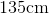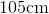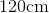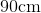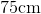Explanation:

To find the perimeter of a hexagon, we will use the following formula: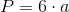where a is the length of one side of the hexagon.

Now, we know the length of one side of the hexagon is 15cm.

Knowing this, we can substitute into the formula.  We get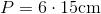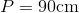### Example Question #3 : Isee Upper Level (Grades 9 12) Mathematics Achievement

Find the perimeter of a hexagon with a side having a length of 13cm.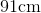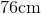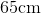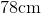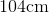Explanation:

A hexagon has 6 sides.  So, to find the perimeter, we will use the following formula: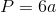where a is the length of one side.

Now, we know a side has a length of 13cm.  Knowing this, we can substitute into the formula.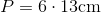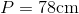### Example Question #4 : Isee Upper Level (Grades 9 12) Mathematics Achievement

A hexagon has a side of length 13cm.  Find the perimeter.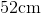Explanation:

To find the perimeter of a hexagon, we will use the following formula:where a is the length of one side of the hexagon.  We multiply it by 6 because a hexagon has 6 equal sides.

So, we know the length of one side is 13cm.  Knowing this, we can substitute into the formula.  We get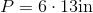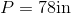### Example Question #5 : Isee Upper Level (Grades 9 12) Mathematics Achievement

Find the perimeter of a hexagon with a side having a length of 12in.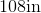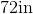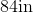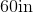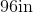Explanation:

To find the perimeter of a hexagon, we will use the following formula:where a is the length of one side of the hexagon.  Because a hexagon has 6 equal sides, we can use any side in the formula.

So, we know the length of one side is 12in.  So, we can substitute.  We get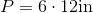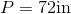### Example Question #6 : Isee Upper Level (Grades 9 12) Mathematics Achievement

Find the perimeter of a hexagon if it contains a side having a length of 17cm.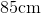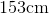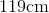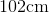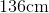Explanation:

To find the perimeter of a hexagon, we will use the following formula:where a is the length of one side of the hexagon.

Now, we know the length of one side of the hexagon is 17cm.

Knowing this, we can substitute into the formula.  We get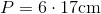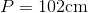### Example Question #11 : Plane Geometry

Find the perimeter of a hexagon with a side having a length of 7cm.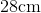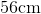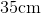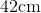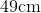Explanation:

A hexagon has 6 equal sides.  To find the perimeter of a hexagon, we will use the following formula:where a is the length of one side of the hexagon.

Now, we know one side has a length of 7cm. So, we get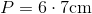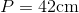### Example Question #12 : Plane Geometry

What is the perimeter of a hexagon with a side length of 1?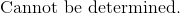Explanation:

A hexagon has 6 similar sides.

The formula to find the perimeter of a hexagon is: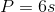Substitute the side into the equation.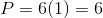The perimeter of the hexagon is.

The answer is: